# 深度学习的数学 (3)：神经网络的思想 1-3(激活函数：将神经元的工作一般化)

《深度学习的数学》基于丰富的图示和具体示例，通俗易懂地介绍了深度学习相关的数学知识。第 1 章介绍神经网络的概况；第 2 章介绍理解神经网络所需的数学基础知识；第 3 章介绍神经网络的 * 优化；第 4 章介绍神经网络和误差反向传播法；第 5 章介绍深度学习和卷积神经网络。书中使用 Excel 进行理论验证，帮助读者直观地体验深度学习的原理。

1 - 2 节中用数学式表示了神经元的工作。本节我们试着将其在数学上一般化。

### 简化神经元的图形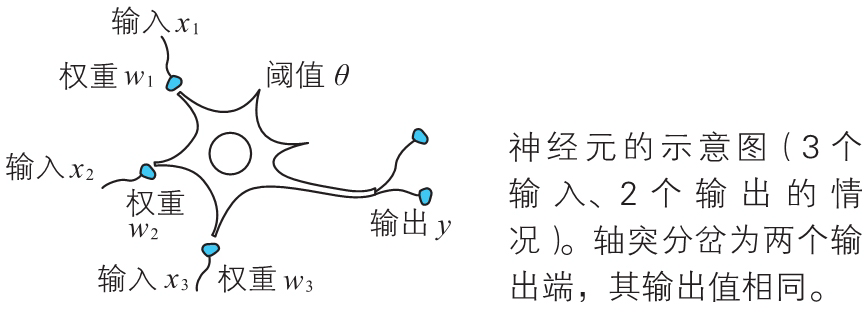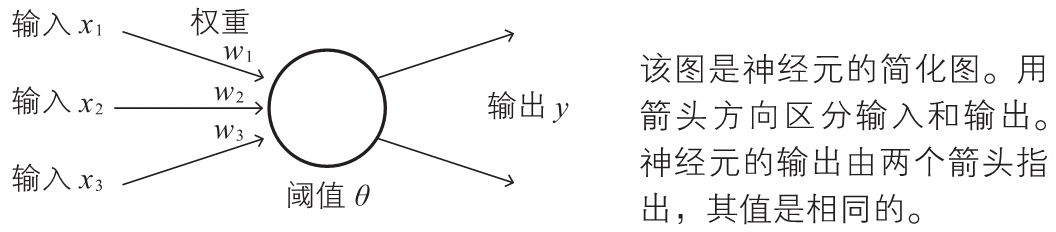### 激活函数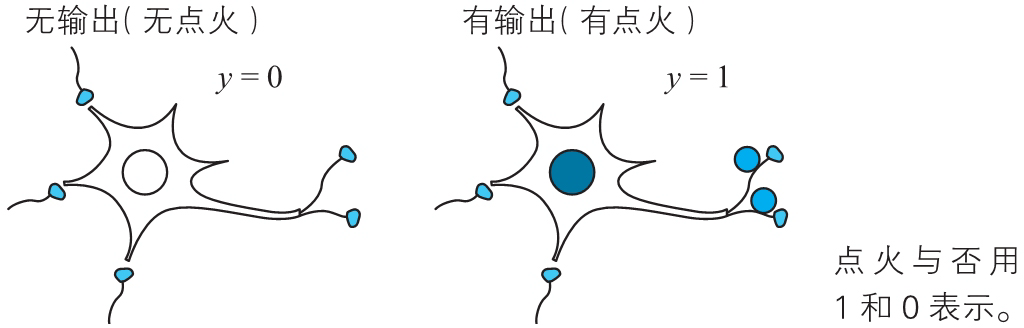$y=a(w_1x_1+w_2x_2+w_3x_3-\theta)\quad\quad\quad\quad\quad\quad\quad\quad(2)$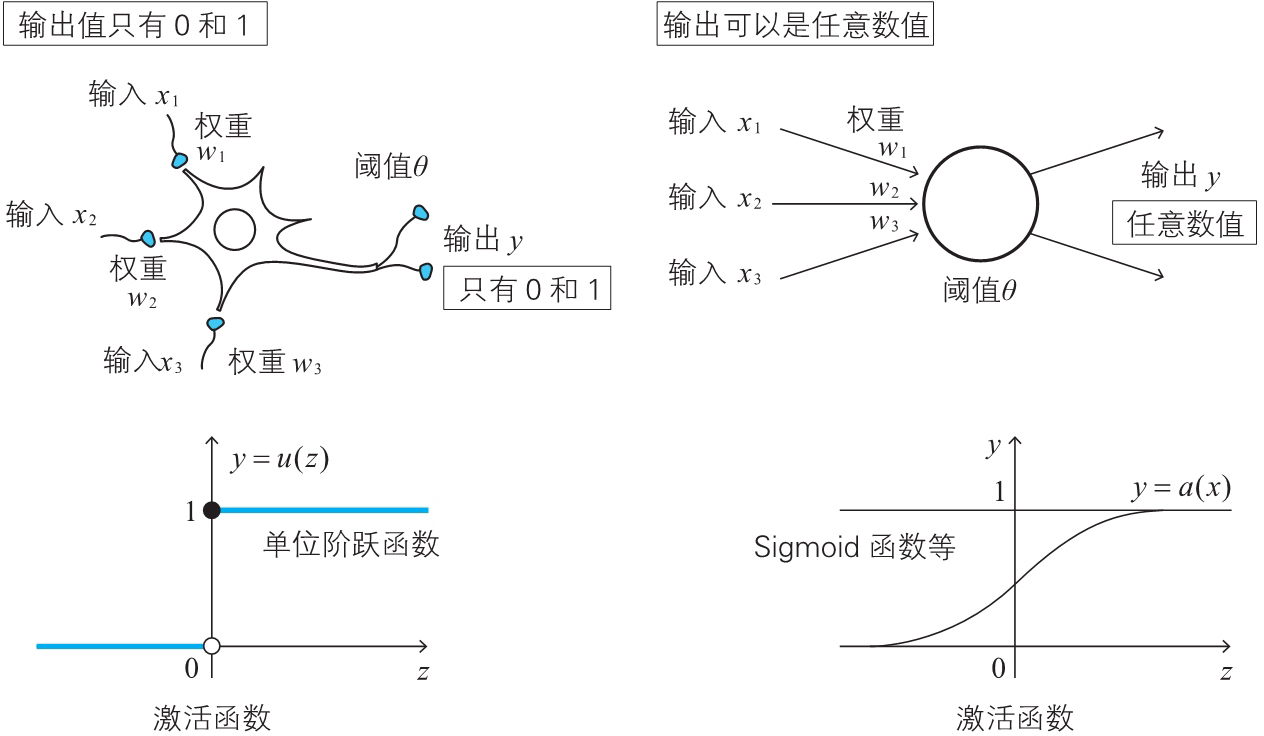### Sigmoid 函数

$\sigma(z)=\frac{1}{1+{\rm e}^{-z}}~~({\rm e}=2.718281\dots)\quad\quad\quad\quad\quad\quad\quad\quad(3)$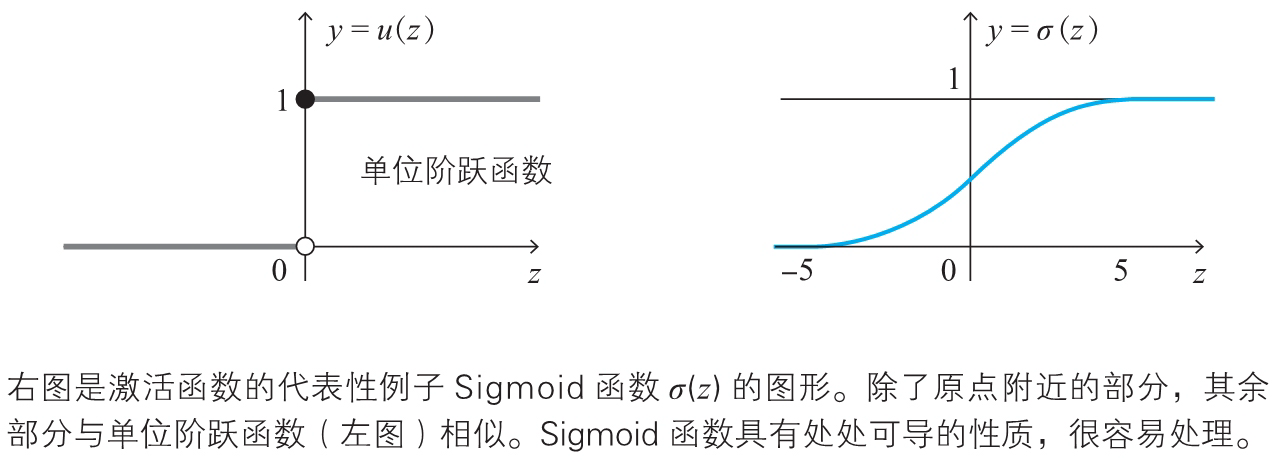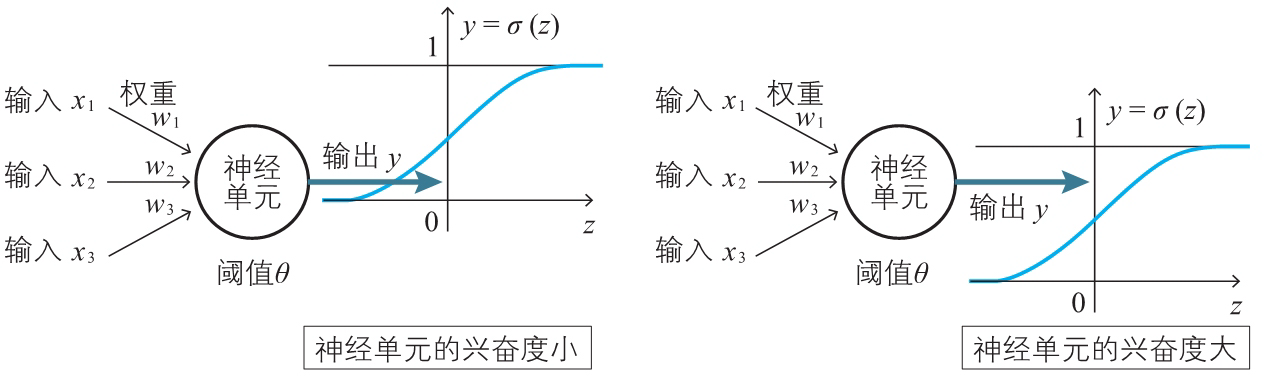### 偏置

$y=a(w_1x_1+w_2x_2+w_3x_3-\theta)\quad\quad\quad\quad\quad\quad\quad\quad(2)$

$y=a(w_1x_1+w_2x_2+w_3x_3+b)\quad\quad\quad\quad\quad\quad\quad\quad(4)$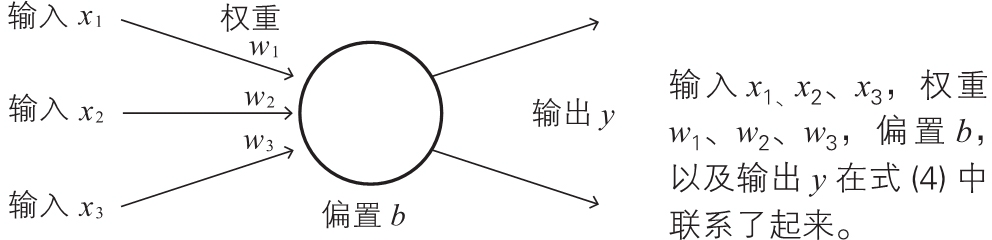$z=w_1x_1+w_2x_2+w_3x_3+b\quad\quad\quad\quad\quad\quad\quad\quad(5)$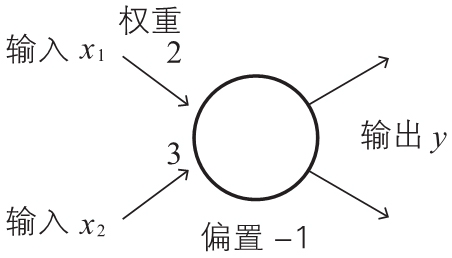0.2 0.1
0.6 0.5

0.2 0.1 2×0.2 + 3×0.1 - 1 = -0.3 0.43
0.6 0.5 2×0.6 + 3×0.5 - 1 = 1.7 0.84

$z=w_1x_x+w_2x_2+w_3x_3+b\times1\quad\quad\quad\quad\quad\quad\quad\quad(6)$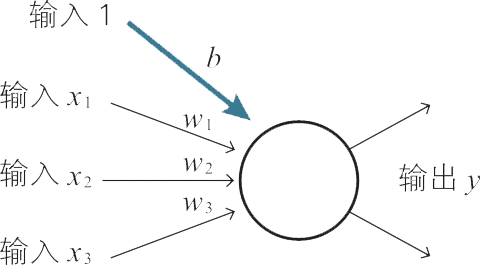$(w_1,w_2,w_3,b)(x_1,x_2,x_3,1)$